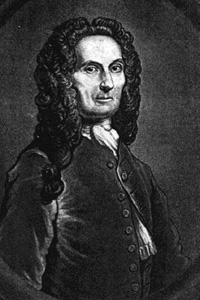A# de Moivre, Abraham (1667–1754)

Abraham de Moivre was a French-born English mathematician who founded analytical trigonometry and stated what has become known as de Moivre's theorem (see below). He also worked on probability theory and the normal distribution, and was a good friend of Isaac Newton. In 1698 he wrote that the theorem had been known to Newton as early as 1676.## de Moivre's theorem

De Moivre's theorem is a theorem that links complex numbers and trigonometry. It states that for any real number x and any integer n,

(cos x + isin x)n = cos(nx) + isin(nx)

By expanding the left hand side and then comparing real and imaginary parts, it is possible to derive useful expressions for cos(nx) and sin(nx) in terms of sin(x) and cos(x). Furthermore, the formula can be used to find explicit expressions for the nth roots of unity: complex numbers z such that zn = 1. It can be derived from (but historically preceded) Euler's identity eix = cos x + i sin x and the exponential law (eix)n = einx.